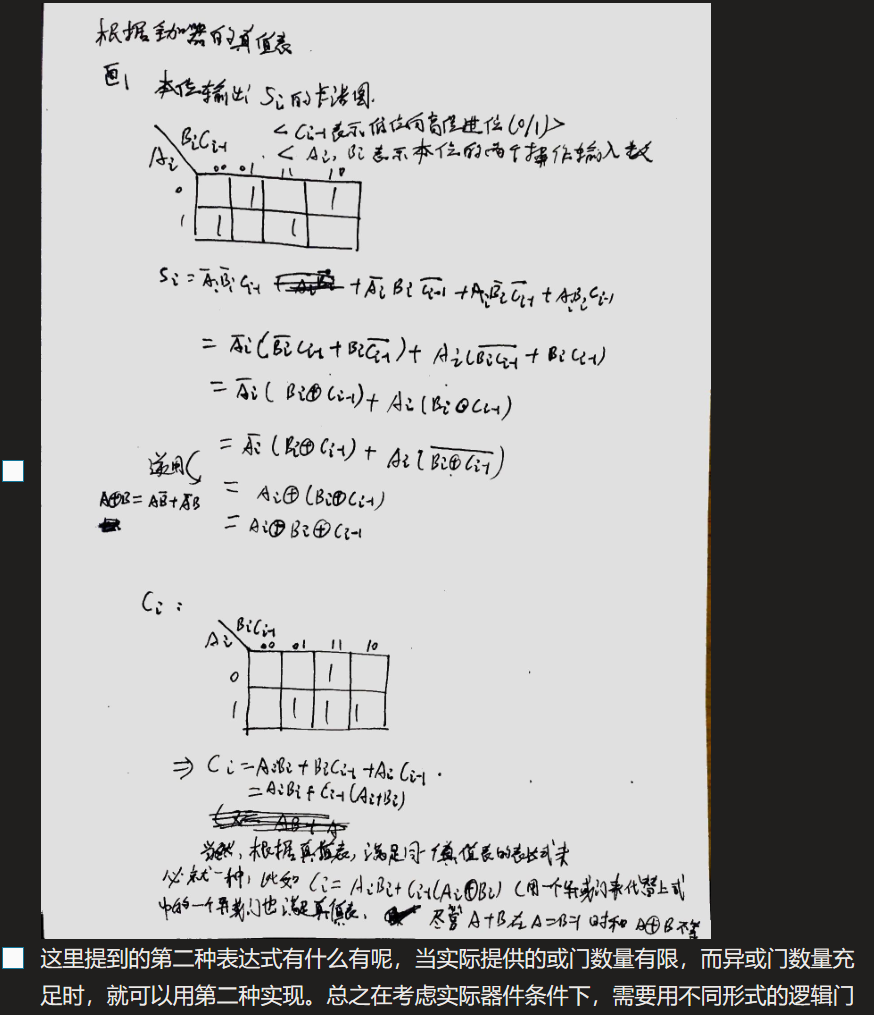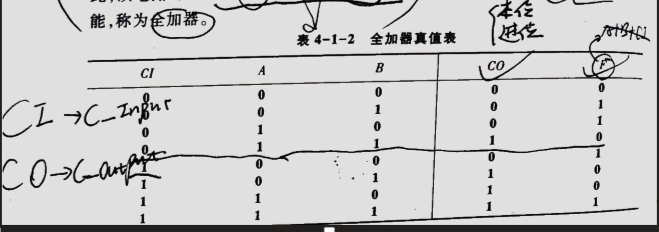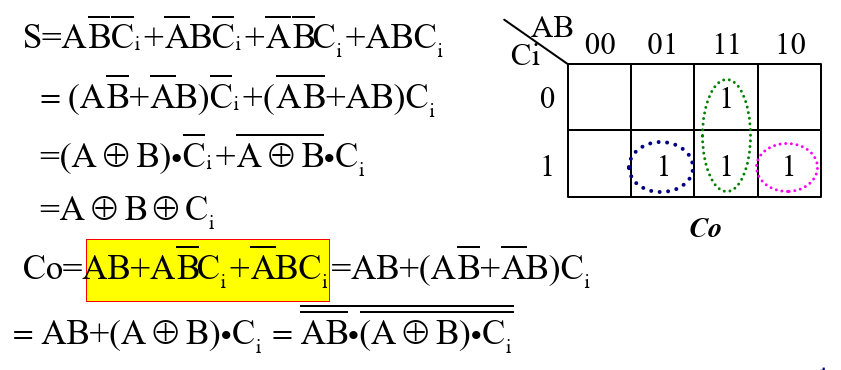• 如果不易最简为目的画如下图所示的卡诺圈,经过推到,可以得到含异或的表达式.如果不易最简为目的画如下图所示的卡诺圈,经过推到,可以得到含异或的表达式.展开全文• 这个处理方法和多项式的处理方法一样。试着写了一下，发现自己对栈的应用能力还是不够，不过很感谢 ... 然后模仿着把这个离散实验作业完成了。.../*程序设计类似与多项式的计算，用双栈来实现，完成变量的表达式
这个处理方法和多项式的处理方法一样。试着写了一下，发现自己对栈的应用能力还是不够，不过很感谢
supermary863的博客http://blog.csdn.net/supermary863/article/details/6363570。

然后模仿着把这个离散实验作业完成了。可能存在一些小问题，但是总体思路就是那样吧。

/*程序设计类似与多项式的计算，用双栈来实现，完成<任意个>变量的表达式的运算*/
/*变量请统一用小写字母*/
/*目前支持的逻辑运算符有~（非），V(析取，用大写字母V)，^(合取，用shitf + 6), 以及->（蕴含）。
没有添加<->（只是多添加一个操作符，优先级同->，同理，有兴趣的读者可以自己实现）*/
/*程序运行方式见main函数入口。*/

#include <iostream>
#include <string>
#include <cstring>
#include <cstdio>
#include <stack>
using namespace std;

class TrueFalseTable
{
protected:
char x;     //存储变量。
int x_num;      //变量数。
int value; //下标对应变量的值0或1。
int Ans;    //每行真值表计算一个值。
/*打印开头部分*/
void printTitle()
{
for(int i = 0; i < x_num; i ++)
{
printf("%c\t", x[i]);
}
printf("-------------------------------------------------\n");
}
/*检测是否穷举了所有的真值表*/
bool check_end()
{
for(int i = 0; i < x_num; i ++)
{
if(value[i] == 0)
return false;
}
return true;
}
/*获得字符对应的当前真值*/
int getValue(char c)
{
for(int i = 0; i < x_num; i ++)
{
if(x[i] == c)
{
return value[i];
}
}
return -1;
}
public:
TrueFalseTable() {Ans = 0;}

/*允许用字符串直接初始化表达式*/
{
Ans = 0;
}

/*初始化，主要是输入表达式，统计变量个数*/
void Init()
{
int tmp = {0}, k = 0;
for (int i = 0; i < read.size(); i ++)
{
{
}
}
for(int i = 0; i < 27; i ++)
{
if(tmp[i] != 0)
{
x[k++] = i + 'a'; //所有出现字母均保存了。
}
}
x_num = k;
memset(value, 0, sizeof(value));
printTitle();
}
/*产生下一组变量的真值*/
bool next_value()
{
if(check_end())
return 0;
value[x_num - 1] ++;
for(int i = x_num-1; i >= 0; i --)
{
if(value[i] > 1)
{
value[i] = 0;
value[i-1] ++;
}
}
return 1;
}
/*输出每一行真值表*/
void printValue()
{
for (int i = 0; i < x_num; i ++)
{
printf("%d\t", value[i]);
}
printf("%d\n", Ans);
}
};

/*因为再写到上面的类就太臃肿了，写一个<子类>
用于计算表达式的值(双栈)*/
class Calculate : public TrueFalseTable
{
private:
stack<int> data;    //数据栈
stack<int> oper;    //符号栈
public:
Calculate() {}
{
}
/*计算表达式的值*/
void calculate()
{
char op = {'~', '>', '^', 'V', '(',')','#'}; //运算符，其中->用>表示。#为栈底。
int  in = {5, 3, 3, 3, 1, 6, 0};            //栈内优先级；
int  out = {4, 2, 2, 2, 6, 1, 0};           //栈外优先级 < 栈内优先级；
int a, b, thera;
oper.push(getIndex('#'));
int i = 0;
int index = oper.top();
while( i < read.size() || op[index] != '#')
{
//遇到表达式中的->， -不读取。
{
i++;
continue;
}

{
i ++;
}

else
{
//遇到~,单独处理。
if(oper.top() == 0)
{
oper.pop();
a = data.top();
data.pop();
a = !a;
data.push(a);
index = oper.top();
continue;
}
//末尾没有符号来比较，那么直接计算。
{
}
else
{
}

{
case '>':
a = data.top();
data.pop();
b = data.top();
data.pop();
thera = oper.top();
oper.pop();
data.push(fiture(b, thera, a));
break;
case '<':
{
i ++;
}
break;
case '=':
oper.pop();
{
i ++;
}
break;
}
}
//字符串最后为一个右括号，就结束。
if(i == read.size() - 1 && oper.top() == 5)
{
oper.pop();
i ++;
}
index = oper.top();
}
Ans = data.top();
data.pop();
oper.pop();
}
private:
/*获得运算符在数组中的下标*/
int getIndex(char c)
{
switch(c)
{
case '~':
return 0;
case '>':
return 1;
case '^':
return 2;
case 'V':
return 3;
case '(':
return 4;
case ')':
return 5;
case '#':
return 6;
default:
return -1;
}
}
/*比较栈内运算符和栈外运算符的优先级*/
char precede(int i1, int i2)
{
//		char op = {'~', '>', '^', 'V', '(',')','#'}; //运算符，其中->用>表示。#为栈底。
int  in = {5, 3, 3, 3, 1, 6, 0};            //栈内优先级；
int  out = {4, 2, 2, 2, 6, 1, 0};           //栈外优先级 < 栈内优先级；
if(in[i1] > out[i2])
{
return '>';
}
else if(in[i1] < out[i2])
{
return '<';
}
else
{
return '=';
}
}

int fiture(int a, int ope, int b)
{
switch(ope)
{
case 0:
return !a;
case 1:
return !a || b;
case 2:
return a && b;
case 3:
return a || b;
}
}
};

int main()
{
Calculate t("(p^~q)V(~p^q)");	//   1、直接在定义类的时候就给表达式。
//    Calculate t;                      //   2、通过控制台输入表达式。
t.Init();
do
{
t.calculate();
t.printValue();
}
while(t.next_value());
return 0;
}



展开全文• ## 由真值表求逻辑表达式的方法

万次阅读 多人点赞 2020-02-12 08:59:59
第一种方法：以真值表内输出端“1”为准 第一步：从真值表内找输出端为“1”的各行,把每行的输入变量写成乘积形式;遇到“0”的输入变量上加非号。...第二步：把各求和项相乘,即得逻辑函数表达式。 总结，哪...
第一种方法：以真值表内输出端“1”为准
第一步：从真值表内找输出端为“1”的各行,把每行的输入变量写成乘积形式;遇到“0”的输入变量上加非号。
第二步：把各乘积项相加,即得逻辑函数的表达式。
第二种方法：以真值表内输出端“0”为准
第一步：从真值表内找输出端为“0”的各行,把每行的输入变量写成求和的形式,遇到“1”的输入变量上加非号。
第二步：把各求和项相乘,即得逻辑函数表达式。
总结，哪种方法得到的表达式简洁就用哪种。
展开全文• 编程实现求解逻辑表达式真值表、主析取范式、主合取范式 一：程序设计思想： 总思想可分为三部分： 1）真值表的求解： 对于一个含n个命题变元的表达式（n为相异的命题变元总个数），其真值表可看作由从0到2ⁿ-1的...
离散数学
编程实现求解逻辑表达式的真值表、主析取范式、主合取范式
一：程序设计思想：
总思想可分为三部分： 1）真值表的求解： 对于一个含n个命题变元的表达式（n为相异的命题变元总个数），其真值表可看作由从0到2ⁿ-1的二进制数的变化表。因此，可以通过按行列举0到2ⁿ-1的二进制数来模拟真值表中命题变元的值。而对于表达式，在每一行，其值可通过运用该行的命题变元的值来求解。为此，应考虑： ①建立命题变元与0/1的键值对关系。可通过C++：stl::map来建立。 ②理解表达式并求值。 2) 表达式求解： 对于表达式，应考虑： ①定义各个运算符的运算法则。 ②定义各个运算符的优先级关系。 求解： 在求解表达式的过程中，可利用两个栈，分别存入临时读取的命题变元的值和逻辑运算符。在依次读取表达式的过程中，通过比较运算符的优先级关系，边读取边运算，并将值存入储存值的栈中。最终，读取完毕，求解完成。储存值的栈的栈顶即为逻辑表达式的值。 3）求解主合取范式、主取范式： 主合取范式： 与1)中思想类似，在输出真值表的过程中，若某行的表达式的值为0，通过该行的map中的键值对关系，按照相应的格式，将命题变元或其否定存入主合取范式的字符串中。 同理， 主析取范式：在输出真值表的过程中，若某行的表达式的值为1，通过该行的map中的键值对关系，按照相应的格式，将命题变元或其否定存入主析取范式的字符串中。 最后，一并输出两个字符串即可。
二：程序源码：
#include<string>
#include<vector>
#include<map>
#include<stack>
#include<iostream>
#include<algorithm>
#include<math.h>

using namespace std;

// fun1： (十进制转换为二进制) 赋真值
void fun1( int x,  map <char,int>& m){
auto p = --m.end();//由于map升序排列，用迭代器p指向map的末尾
for(int i = 1; i <= distance( m.begin(), m.end()); i ++){
p->second = x % 2;
x = x / 2;
p --;
}
}

//compare：用于描述运算符之间的优先级关系
int compare( char a, char b){
if(a == '!') {
if( b == '(') return -1;
else if(b == '!') return 0;
else return 1;
}

else if(a == '@' ){
if((b == '(' || b == '!')) return -1;
else return 1;
}

else if(a == '#') {
if((b == '@'|| b == '(' || b == '!')) return -1;
else return 1;
}
else if(a == '$' ){ if((b == '@'|| b == '(' || b == '#' || b == '!')) return -1; else return 1; } else if(a == '%'){ if(( b == '%' || b == ')')) return 1; else return -1; } else if(a == '(' ){ if(b == ')') return 0; else return -1; } } //value：定义运算法则 并计算 a ? b 并返回值 int value( int a, char c, int b){ if(c == '@') return a*b;//合取 if(c == '#') { if(a == 0 && b == 0) return 0; else return 1; }//析取 if(c == '$') {
if(a == 1 && b == 0) return 0;
else return 1;
}//条件

if(c == '%') {
if(a == b) return 1;
else return 0;
}//双条件
}

//key：表达式求解 利用两个栈分别存储 值和运算符 逐渐求解
int key(string s, map <char,int> m){
s += ")";
stack <int> s1;
stack <char> s2;	s2.push('(');
for(int i = 0; i != s.length(); i ++){
if(isalpha(s[i])){
auto p = m.find(s[i]);
s1.push(p->second);
}
else if(isdigit(s[i])) s1.push(s[i] - '0');
else {
if(compare( s2.top(), s[i]) == -1) s2.push(s[i]);
else if(compare( s2.top(), s[i]) == 0) s2.pop();
else if(compare( s2.top(), s[i]) == 1) {
if(s2.top() == '!') {
int a = s1.top();	s1.pop();
if(a == 1) s1.push( 0);
else s1.push( 1);

s2.pop();	i --;
}
else{
int b = s1.top(); s1.pop();
int a = s1.top(); s1.pop();
s1.push( value( a, s2.top(), b));
s2.pop();	s2.push( s[i]);
}
}
}

}
return s1.top();
}

//fun2：(利用真值表) 计算主合取范式和主析取范式 并存入s1, s2
void fun2(int i, int key, map <char,int> m, vector <int>& Max,vector <int>& min, string& s1, string& s2){
if(key == 0) {
Max.push_back(i);
s1+='(';
for(auto i = m.begin(); i != m.end(); i ++){
if(i->second == 1) s1+='!';
s1+=i->first;
if(i != --m.end()) s1+='#';
}
s1+=')';
s1+='@';
}
else {
min.push_back(i);
s2+='(';
for(auto i = m.begin(); i != m.end(); i ++){
if(i->second == 0) s2+='!';
s2+=i->first;
if(i != --m.end()) s2+='@';
}
s2+=')';
s2+='#';
}
}

//print函数: 输出 主合取范式和主析取范式
void print( vector <int> Max, vector <int> min, string s1, string s2){

printf("主合取范式：\n");
cout << "∏";
for(auto i = Max.begin(); i < Max.end(); i ++){
if( i == Max.begin()) {
cout << *i;
}
else cout << ", " << *i;
}
cout << endl;

for(int i = 0; i < s1.length(); i++){
if(i == s1.length() - 1) {
if(s1[i] != '@') cout << s1[i];
}
else cout << s1[i];
}
cout<< endl<< endl;

printf("主析取范式：\n");
cout << "∑";
for(auto i = min.begin(); i < min.end(); i ++){
if( i == min.begin()) {
cout << *i;
}
else cout << ", " << *i;
}
cout << endl;

for(int i = 0; i < s2.length(); i++){
if(i == s2.length() - 1) {
if(s2[i] != '#') cout << s2[i];
}
else cout << s2[i];
}
}

int main(){

//声明
printf("由于合取、析取等特殊字符难以表示，故作以下声明\n");
printf("声明:\n");
printf("以!代替否定\n");
printf("以@代替合取\n");
printf("以#代替析取\n");
printf("以\$代替条件\n");
printf("以%代替双条件\n\n");
printf("支持使用括号()、支持n个命题变元、支持重复的命题变元、支持0/1参与运算\n\n");
printf("请输入合法的表达式:\n->");

string s;//表达式
string s1, s2;// 合取范式和主析取范式
vector <int> Max, min;//集合：存储大项和小项的下标
map < char, int> m;// map<char, int>: 建立命题变元与 0/1 的键值对关系
cin >> s;
cout << endl;

//map: P Q R ... ...  map的初始化
for(auto i = s.begin(); i != s.end(); i ++){
if(isalpha(*i)) m.insert(make_pair( *i, 0));
}

printf("真值表：\n");

//输出真值表——表头
//1 输出 键 map_key
for(auto i = m.begin(); i != m.end(); i ++){
cout << i->first << "   ";
}

//2 输出表达式
cout << s;
cout << endl;

int n = pow( 2,distance(m.begin(), m.end()));//总情况个数

//（按行依次）输出真值表——真值
for(int i = 0; i < n; i ++){

//1 为键赋值
fun1( i, m);

//2 输出 值 map_value
for(auto i = m.begin(); i != m.end(); i ++)
cout << i->second << "   ";

//3 计算并输出表达式的真值
cout << key( s, m) << endl;

//4 计算主合取范式和主析取范式 并存入s1, s2
fun2( i, key( s, m), m, Max, min, s1, s2);
}

cout << endl << endl;

print( Max, min, s1, s2);//输出 主合取范式和主析取范式

return 0; // 程序结束
}


展开全文c++ 开发语言 后端
• 真值表表达式的化简，想起来似乎不好弄，其实很简单，三步就好： 1：找所有真值为1的情况。 2：用“与”组织输出为1的组合 3：将所有组合用“或”结合 举例： 表达式为： 转载于:...
• 【2018.6.2】今天在看书的时候无意中需要使用... 据说有两种方法，这里都列举一下吧： 方法一： step 1 首先，根据真值表当中结果是true的项，找出它的两个输入项，然后进行与运算；如果遇到输入项的取值是fals...
• 根据这张真值表，我们还需要将其转化成逻辑函数，即用电路的逻辑语言来描述输出量与输入量之间的关系，替代这一张庞大的真值表。只有明确地表示出输出与输入之间对应的函数关系或者功能模块的逻辑...
• ## python - 根据表达式打印真值表

千次阅读 多人点赞 2018-11-03 20:31:34
输入逻辑表达式，输出真值表，支持六个基本逻辑运算 最终效果：  输入合适公式（没有考虑优先级，只根据括号优先），输出时会提取其中的元素（比如这里有A B C），并打印真值表。  算法思路：  求值的一般...python 离散数学 递归 set
• 文章目录1. 与门2. 或门3. 非门4. 异或门5. 同或门6. 与非门7. 或非门 1. 与门 2. 或门 3. 非门 4. 异或门 5. 同或门 6. 与非门 7. 或非门数字信号处理
• 要求化简的最终结果 是逻辑函数的标准形式，即用最大项、最小项表示的表达式 一、代数转换法（要求对公理定理熟悉掌握） 化简成 与或表达式 化简到最后 缺省变量的项用 X = X（Y+Y非）扩充变量 将上面逻辑函数...
• 从布尔函数真值表求ANF 布尔函数简介 在密码学中，布尔函数是加密算法的核心部件之一。 布尔函数指从 F2n\mathbb{F}_2^nF2n​ 到 F2\mathbb{F}_2F2​ 的映射。 简单举例如下： 一个三元(n=3n=3n=3)布尔函数 FFF 是...
• 逻辑功能中简单概括得出的逻辑函数，往往不是最简表达式，根据这样的非最简式来实现电路，系统会过于复杂，成本过高，同时，电路运行的安全性和可靠性也无法得到保障。 为了降低系统成本，提高工作可靠性，应在不...数字电子技术 函数
• 实验一 命题逻辑：求给定命题公式的真值表 实验原理：[可忽略] 将命题公式A在所有赋值下取值情况列成表，称作A的真值表。 构造真值表的具体步骤如下： (1) 找出公式中所含的全体命题变项p1,p2,…,pn (若无下角标就...编程语言 c语言 数学 程序设计
• 全加器的真值表 全加器的代数表达 和S的推导： 由真值表可写出 S=A‾ B‾ C+A‾ B C‾+AB‾ C‾+ABC S =\overline A\ \overline B \ C+\overline A \ B \ \overline C + A \overline B \...
• 1，逻辑真值表 2，逻辑函数式：由与或非等各种运算符所构成的逻辑表达式...2.真值表逻辑函数式：根据给定真值表，先找出Y的值为1的部分以及其对应的输入组合，然后把这些输入组合分别写成乘积项，值为1的写成原变...
• 4变量卡诺图化简软件 根据真值表自动化简成最简与或表达式 电子技术基础 数字部分 课程设计 作业卡诺图化简
• 实验仪器Multisim电路仿真74LS00 二输入端四与非门 3片 74LA86 二输入端四异或门 1片 74LS54 四组输入与或非门 1片实验目的掌握组合逻辑电路的功能测试。 验证半加器和全加器的逻辑功能。 学会逻辑分析仪的使用方法...
• 在本学期的数字电路学习过程中，我们需要根据给定的逻辑电路或者逻辑表达式，列出真值表。倘若输入逻辑变量有多个，手写无疑是非常麻烦的。就算敲键盘一个一个输入到Excel在运算也没快到哪去。接下来用一个例子给...matlab
• 逻辑表达式和关系表达式 0表示假，1表示，任何非0的数为 逻辑表达式是指运算符为或||、与&amp;&amp;、非！的表达式。返回值为0或1，0表示false，非0表示true. 例如!0返回1，0&amp;&amp;1返回0...
• 首先创建一个类，利用python自身的优势对表达式进行解析 # &为合取，v为析取，~为非，>为条件联结词，*为双条件联结词 class Variable: def __init__(self, value=0): self.value = value # 返回两个...python 递归 运算符
• 问题1：一位全加器 assign sum = a ^ b ^ cin; assign cout = a&b | a&cin | b&cin; 一位全加器，sum为输入加进位位异或（即1的个数为奇数时，sum==1...不过现在FPGA基本是四输入查找，所以直接a&...
• 逻辑函数表达式的形式 逻辑代数是研究逻辑变量及其相互关系的一门科学，由于它是英国数学家乔治·布尔（Geoge Boolen）于 1849 年提出来的，因此也称为布尔代数。逻辑代数是分析和设计数字电路的基础。电子...
• 文章目录前言一、根据简化真值表绘制电路二、做法1.相关概念2.步骤总结 前言 ...2.根据简化真值表写出逻辑函数的与或表达式； 3.普通代数变量与逻辑变量的差异； 4.关系表达式与逻辑表达式的区别.
• 一个逻辑函数可以用真值表、表达式、逻辑图、波形图 等方法来表示。既然它们都是表示同一种逻辑关系，显然可以 互相转换 。 由真值表写逻辑式 由表达式画逻辑图 由函数表达式求真值表 已知逻辑图写逻辑表达式 由...
• PAGE PAGE 5 第4章 组合逻辑电路 41 分析下图所示电路的逻辑功能写出输出的逻辑表达式列出真值表说明其逻辑功能 经过真值表分析其逻辑功能为当ABC三个输入信号中有且只有两个为1时输出为1其他为0 42 逻辑电路如下图...
• 函数可以通过声明定义，也可以是一个表达式函数声明在之前的教程中，你已经了解了函数声明的语法 :function functionName(parameters) { 执行的代码 }函数仅仅是声明之后,它是不可能立即执行的，还要等到 在我们...JS JavaScript 闭包 this
• 图2-3展示了用于实现逻辑电路的符号和逻辑门的真值表。与（AND）门、或（OR）门、与非（NAND）门、或非（NOR）门、非（NOT）门在第1章中有过讨论。异或（XOR）门、同或（XNOR）门是有两个输入的逻辑门。当两个输入...
• 前言：恰逢双十一，在京东买书满一百减五十（不买立减100%^^），买了...文章目录一、FPGA的概念（P1）二、逻辑代数的构成（P1）三、逻辑代数的定理（P2）四、逻辑表达式（P3）五、真值表（P3）六、运算逻辑门（P4）...FPGA
• 我昨天听这节课的时候，发现这次的内容和离散数学里面的知识几乎是一样的诶！...逻辑函数表达式的基本形式 “与-或”表达式 由若干“与项”进行“或”运算构成的表达式 “与项”可以是单个变量的原变量，可
• 1.真值表法 2.拆项法 3.卡诺图法 1.拆项法 以最小项表达式为例 F=AC+非AB+BC 第一项不含B，所以给第一项与上（B+非B） 第二项不含C，所以给第二项与上（非C+C） 第二项不含A，所以给第二项与上（非A+A） ......